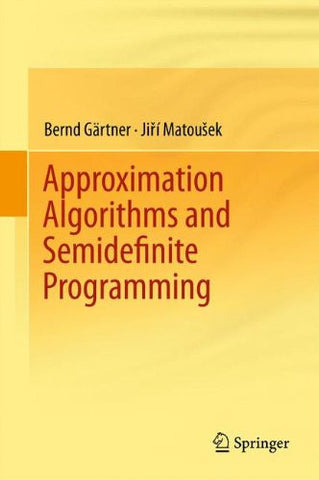Approximation Algorithms and Semidefinite Programming

• \$97.19
• Save \$15
• Binding: Hardcover
• Author: Bernd GÃ¤rtner;Jiri Matousek
• Publish Date: 2012-01-10

Attention : For textbook, access codes and supplements are not guaranteed with used items.

Semidefinite programs constitute one of the largest classes of optimization problems that can be solved with reasonable efficiency - both in theory and practice. They play a key role in a variety of research areas, such as combinatorial optimization, approximation algorithms, computational complexity, graph theory, geometry, real algebraic geometry and quantum computing. This book is an introduction to selected aspects of semidefinite programming and its use in approximation algorithms. It covers the basics but also a significant amount of recent and more advanced material.Â Â

There are many computational problems, such as MAXCUT, for which one cannot reasonably expect to obtain an exact solution efficiently, and in such case, one has to settle for approximate solutions. For MAXCUT and its relatives, exciting recent results suggest that semidefinite programming is probably the ultimate tool. Indeed, assuming the Unique Games Conjecture, a plausible but as yet unproven hypothesis, it was shown that for these problems, known algorithms based on semidefinite programming deliver the best possible approximation ratios among all polynomial-time algorithms.

Â

This book follows the âsemidefinite sideâ of these developments, presenting some of the main ideas behind approximation algorithms based on semidefinite programming. It develops the basic theory of semidefinite programming, presents one of the known efficient algorithms in detail, and describes the principles of some others. It also includes applications, focusing on approximation algorithms.